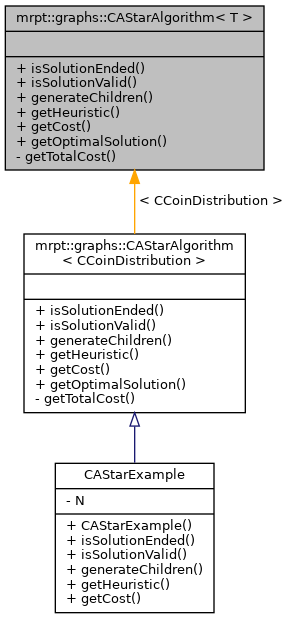MRPT  1.9.9
mrpt::graphs::CAStarAlgorithm< T > Class Template Referenceabstract

Detailed Description

template<typename T> class mrpt::graphs::CAStarAlgorithm< T >

This class is intended to efficiently solve graph-search problems using heuristics to determine the best path.

To use it, a solution class must be defined so that it contains all the information about any partial or complete solution. Then, a class inheriting from CAStarAlgorithm<Solution class> must also be implemented, overriding five virtual methods which define the behaviour of the solutions. These methods are isSolutionEnded, isSolutionValid, generateChildren, getHeuristic and getCost. Once both classes are generated, each object of the class inheriting from CAStarAlgorithm represents a problem who can be solved by calling getOptimalSolution. See http://en.wikipedia.org/wiki/A*_search_algorithm for details about how this algorithm works.

CAStarAlgorithm::isSolutionEnded
CAStarAlgorithm::isSolutionValid
CAStarAlgorithm::generateChildren
CAStarAlgorithm::getHeuristic
CAStarAlgorithm::getCost

Definition at line 39 of file CAStarAlgorithm.h.

#include <mrpt/graphs/CAStarAlgorithm.h>

Inheritance diagram for mrpt::graphs::CAStarAlgorithm< T >:Public Member Functions

virtual bool isSolutionEnded (const T &sol)=0
Client code must implement this method. More...

virtual bool isSolutionValid (const T &sol)=0
Client code must implement this method. More...

virtual void generateChildren (const T &sol, std::vector< T > &sols)=0
Client code must implement this method. More...

virtual double getHeuristic (const T &sol)=0
Client code must implement this method. More...

virtual double getCost (const T &sol)=0
Client code must implement this method. More...

int getOptimalSolution (const T &initialSol, T &finalSol, double upperLevel=HUGE_VAL, double maxComputationTime=HUGE_VAL)
Finds the optimal solution for a problem, using the A* algorithm. More...

Private Member Functions

double getTotalCost (const T &sol)
Calculates the total cost (known+estimated) of a solution. More...

◆ generateChildren()

template<typename T>
 virtual void mrpt::graphs::CAStarAlgorithm< T >::generateChildren ( const T & sol, std::vector< T > & sols )
pure virtual

Client code must implement this method.

Given a partial solution, returns all its children solution, regardless of their validity or completeness.

Implemented in CAStarExample.

◆ getCost()

template<typename T>
 virtual double mrpt::graphs::CAStarAlgorithm< T >::getCost ( const T & sol )
pure virtual

Client code must implement this method.

Given a (possibly partial) solution, calculates its cost so far. This cost must not decrease with each step. That is, a solution cannot have a smaller cost than the previous one from which it was generated.

Implemented in CAStarExample.

◆ getHeuristic()

template<typename T>
 virtual double mrpt::graphs::CAStarAlgorithm< T >::getHeuristic ( const T & sol )
pure virtual

Client code must implement this method.

Given a partial solution, estimates the cost of the remaining (unknown) part. This cost must always be greater or equal to zero, and not greater than the actual cost. Thus, must be 0 if the solution is complete.

Implemented in CAStarExample.

◆ getOptimalSolution()

template<typename T>
 int mrpt::graphs::CAStarAlgorithm< T >::getOptimalSolution ( const T & initialSol, T & finalSol, double upperLevel = HUGE_VAL, double maxComputationTime = HUGE_VAL )
inline

Finds the optimal solution for a problem, using the A* algorithm.

Returns whether an optimal solution was actually found. Returns 0 if no solution was found, 1 if an optimal solution was found and 2 if a (possibly suboptimal) solution was found but the time lapse ended.

Definition at line 92 of file CAStarAlgorithm.h.

◆ getTotalCost()

template<typename T>
 double mrpt::graphs::CAStarAlgorithm< T >::getTotalCost ( const T & sol )
inlineprivate

Calculates the total cost (known+estimated) of a solution.

Definition at line 79 of file CAStarAlgorithm.h.

◆ isSolutionEnded()

template<typename T>
 virtual bool mrpt::graphs::CAStarAlgorithm< T >::isSolutionEnded ( const T & sol )
pure virtual

Client code must implement this method.

Returns true if the given solution is complete.

Implemented in CAStarExample.

◆ isSolutionValid()

template<typename T>
 virtual bool mrpt::graphs::CAStarAlgorithm< T >::isSolutionValid ( const T & sol )
pure virtual

Client code must implement this method.

Returns true if the given solution is acceptable, that is, doesn't violate the problem logic.

Implemented in CAStarExample.

 Page generated by Doxygen 1.8.14 for MRPT 1.9.9 Git: 7d5e6d718 Fri Aug 24 01:51:28 2018 +0200 at lun nov 2 08:35:50 CET 2020